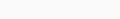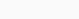# Coursera machine learning week 4 Quiz answer Neural Networks Representation | Andrew NG

In this article, you will find Coursera machine learning week 4 Quiz answer Neural Networks Representation | Andrew NG.  Use “Ctrl+F” To Find Any Questions or Answers. For Mobile Users, You Just Need To Click On Three dots In Your Browser & You Will Get A “Find” Option There. Use These Options to Get Any Random Questions Answer.
Try to solve all the assignments by yourself first, but if you get stuck somewhere then feel free to browse the code. Don’t just copy-paste the code for the sake of completion.  Even if you copy the code, make sure you understand the code first.### Coursera machine learning week 4 Quiz answer Neural Networks Representation | Andrew NG

1. Which of the following statements are true? Check all that apply.

•  Any logical function over binary-valued (0 or 1) inputs x1 and x2 can be (approximately) represented using some neural network.
•  Suppose you have a multi-class classification problem with three classes, trained with a 3 layer network. Let  be the activation of the first output unit, and similarly  and . Then for any input x, it must be the case that .
• A two layer (one input layer, one output layer; no hidden layer) neural network can represent the XOR function.
• The activation values of the hidden units in a neural network, with the sigmoid activation function applied at every layer, are always in the range (0, 1).

2. Consider the following neural network which takes two binary-valued inputs
and outputs. Which of the following logical functions does it (approximately) compute?•  AND

This network outputs approximately 1 only when both inputs are 1.

•  NAND (meaning “NOT AND”)
• OR
•  XOR (exclusive OR)

2. Consider the following neural network which takes two binary-valued inputs
and outputs. Which of the following logical functions does it (approximately) compute?•  AND
•  NAND (meaning “NOT AND”)
•  OR

This network outputs approximately 1 when atleast one input is 1.

•  XOR (exclusive OR)

3. Consider the neural network given below. Which of the following equations correctly computes the activation ? Note:is the sigmoid activation
function.•

Thiscorrectly uses the first row of  and includes the “+1” term of .

•
•
•

4. You have the following neural network:You’d like to compute the activations of the hidden layer . One way to do
so is the following Octave code:You want to have a vectorized implementation of this (i.e., one that does not use for loops). Which of the following implementations correctly compute ? Check all
that apply.

•  z = Theta1 * x; a2 = sigmoid (z);

This version computes  correctly in two steps , first the multiplication and then the sigmoid activation.

•  a2 = sigmoid (x * Theta1);
•  a2 = sigmoid (Theta2 * x);
•  z = sigmoid(x); a2 = sigmoid (Theta1 * z);

5. You are using the neural network pictured below and have learned the parameters  (used to compute ) and  (used to compute  as a function of ). Suppose you swap the parameters for the first hidden layer between its two units so  and also swap the output layer so . How will this change the value of the output?•  It will stay the same.

Swapping  swaps the hidden layers output . But the swap of  cancels out the change, so the output will remain unchanged.

•  It will increase.
• It will decrease
•  Insufficient information to tell: it may increase or decrease.

Disclaimer:  Hopefully, this article will be useful for you to find all the Coursera machine learning week 4 Quiz answer Neural Networks Representation | Andrew NG and grab some premium knowledge with less effort.
Finally, we are now, in the end, I just want to conclude some important message for you, Feel free to ask doubts in the comment section. I will try my best to answer it. If you find this helpful by any means like, comment, and share the post. Please share our posts on social media platforms and also suggest to your friends to Join Our Groups. Don’t forget to subscribe. This is the simplest way to encourage me to keep doing such work.

### FAQs

Is Andrew Ng’s Machine Learning course good?
It is the Best Course for Supervised Machine Learning! Andrew Ng Sir has been like always has such important & difficult concepts of Supervised ML with such ease and great examples, Just amazing!
How do I get answers to coursera assignment?
Use “Ctrl+F” To Find Any Questions Answered. & For Mobile Users, You Just Need To Click On Three dots In Your Browser & You Will Get A “Find” Option There. Use These Options to Get Any Random Questions Answer.
How long does it take to finish coursera Machine Learning?
this specialization requires approximately 3 months with 75 hours of materials to complete, and I finished it in 3 weeks and spent an additional 1 week reviewing the whole course.
How do you submit assignments on Coursera Machine Learning?
Submit a programming assignment Open the assignment page for the assignment you want to submit. Read the assignment instructions and download any starter files. Finish the coding tasks in your local coding environment. Check the starter files and instructions when you need to. Reference

Sharing Is Caring